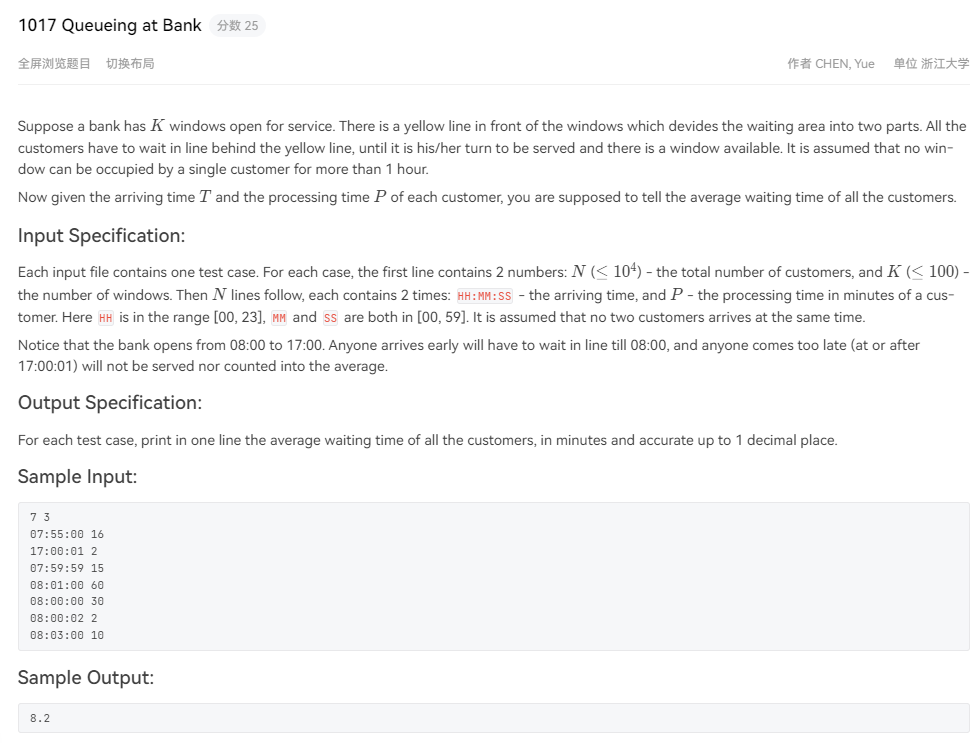# 题目# 解题思路

• 首先本题依旧是把时间都换算成统一单位的，本题涉及到了秒，那就把所有的时间都换算成秒，以0时：0分：0秒为基准，将客户到达的时间业务处理时间都换算成以秒为单位。
• 本题存在所有客户均在17点后来办理业务的情况，那么这样就特别处理，输出`0.0`即可，同时我们不会把17点后来办理业务的客户存入map，所以可以用用map.size()来判断。

# 实现代码

``````#include<iostream>
#include<cstdio>
#include<cstdlib>
#include<map>
#include<algorithm>
#include<vector>
#include<queue>
#include<string>
using namespace std;

int main() {
#ifdef ONLINE_JUDGE
#else
freopen("1.txt", "r", stdin);
#endif

int N,K;
cin>>N>>K;

map<int,int> mmap;
int hour,minute,second,deal_time,sum_time;

for(int i=0;i<N;i++)
{
scanf("%d:%d:%d %d",&hour,&minute,&second,&deal_time);

sum_time=hour * 60 * 60 + minute * 60 + second;     //将时间换算成秒

if(sum_time>61200)
continue;
mmap[sum_time]=deal_time*60;       //依然换算成秒
}

priority_queue<int,vector<int>,greater<int>> Q;
for(int i=0;i<K;i++)
Q.push(28800);

map<int,int>::iterator iter;
double total=0.0;
for(iter=mmap.begin() ; iter!=mmap.end() ; iter++)
{
if(iter->first<Q.top())
{
//说明你来早了，不一定是8点前，有可能是当前的柜台满了，也是来早了
total+=(Q.top() - iter->first);
Q.push(Q.top() + iter->second);
Q.pop();
}
else
{
Q.push(iter->first + iter->second);
Q.pop();
}
}

(!mmap.size())? printf("0.0"):printf("%.1f",(total/60)/mmap.size());

return 0;
}``````

lionの金库